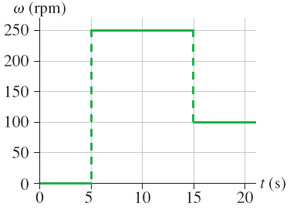# Problem: The angular velocity (in rpm) of the blade of a blender is given in the figure.a. If θ=0rad at t=0s, what is the blade's angular position at t=20s?b. At what time has the blade completed 25 full revolutions?

###### FREE Expert Solution

(a)

Total angle covered is equal to area under the curve on the ω-t graph:

θ = (250rev/min(1min/60s))(15 - 10) + (100rev/min(1min/60s))(20 - 15) = 50 rev (2π rad/rev) = 314 rad

81% (264 ratings)###### Problem Details

The angular velocity (in rpm) of the blade of a blender is given in the figure.

a. If θ=0rad at t=0s, what is the blade's angular position at t=20s?

b. At what time has the blade completed 25 full revolutions?